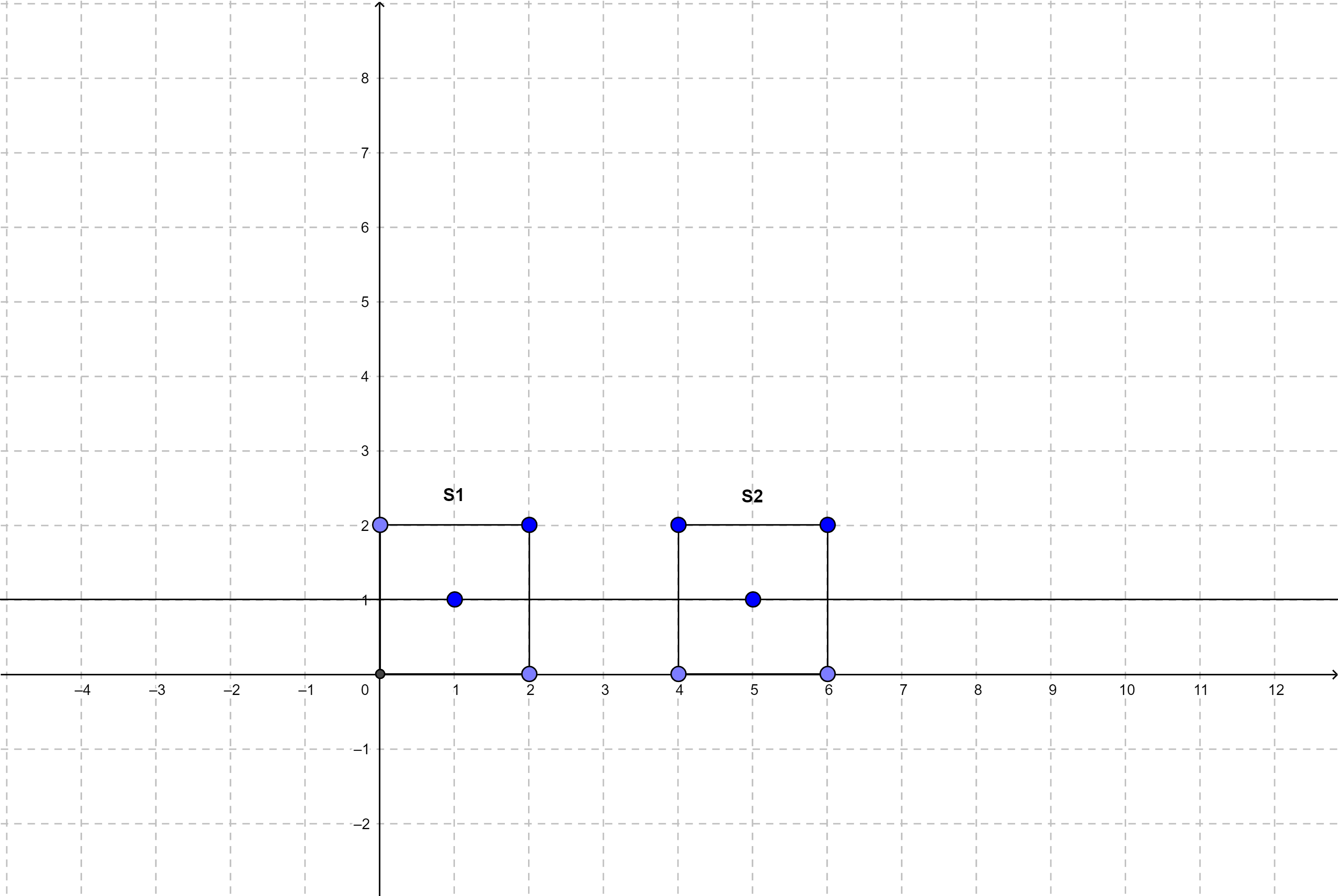# Two Squares

Posted: 26 Feb, 2021
Difficulty: Moderate

## PROBLEM STATEMENT

#### Each square is represented by an array of four points, where each point has two integers denoting the x-coordinate and the y-coordinate, respectively. So, your task is to return an array of two numbers of type double, where the first number represents the slope, and the second number represents the y-intercept of the line. If such a line doesn’t exist, or multiple such lines exist, then return {-1, -1}. If the line is parallel to the Y-axis, then return {0,0}.

##### For Example:
``````If square s1 = {{0, 2}, {2, 2}, {2, 0}, {0, 0}} and square s2 = {{4, 2}, {6, 2}, {6, 0}, {4, 0}}.
````````````So, the two squares are shown in the above figure. The line that would cut these two squares in half has the equation y = 1. So the slope of the line is 0, and the y-intercept is 1. So, we return {0, 1} as the answer.
``````
##### Input format:
``````The first line contains an integer ‘T', which denotes the number of test cases or queries to be run. Then, the T test cases follow.

Four lines follow. Each line contains two space-separated integers, denoting the x and y-coordinates of vertices of the square s1 in clockwise order starting from the top left corner.

Then the next four lines follow. Each line contains two space-separated integers, denoting the x and y-coordinates of vertices of the square s2 in clockwise order.
For more clarity, please refer to the sample inputs.
``````
##### Output format:
``````For each test case, print a single line containing two space-separated numbers of type doubles denoting the slope and the y-intercept of the line, respectively, that would cut the squares in exactly half.

Your answer will be considered correct if the absolute or relative error of the numbers doesn’t exceed 10^-6.

The output for each test case will be printed in a separate line.
``````
##### Note:
``````You do not need to print anything. It has already been taken care of. Just implement the given function.
``````
##### Constraints:
``````1 <= T <= 5000
-100 <= x-coordinate, y-coordinate of both squares <= 100

Time limit: 1 second
``````Approach 1

Approach:

The line that bisects a square must pass through its centre. As there are two squares given in the problem, find the centre of both the squares. The line that passes through both these points is the desired answer. Note that there can be only one possible line that passes through both points. However, if the centre of both squares is the same point, then there can be infinitely many possible lines. So, we return {0, 0} in this case.

Steps:

1. Create four variables of type double to store the coordinates of the centres of the two squares. Let’s name them s1x, s1y, s2x, and s2y. Here s1x and s1y store the x and the y-coordinate of the centre of the square s1. Similarly, s2x and s2y store the x and the y-coordinate of the centre of the square s2.
2. s1x = (s1 + s1 + s1 + s1) / 4.
3. s1y = (s1 + s1 + s1 + s1) / 4.
4. s2x = (s2 + s2 + s2 + s2) / 4.
5. s2y = (s2 + s2 + s2 + s2) / 4.
6. If s1x == s2x and s1y == s2y:
1. This means both the squares have the same centre. So we return {-1, -1} in this case as there can be multiple possible lines.
7. Else if, s1x == s2x and s1y != s2y:
1. This means the line is parallel to the Y-axis. So we return {0, 0}.
8. Create two variables of double date type. Let’s name them slope and intercept.
9. The slope can be calculated as, slope = (s2y - s1y) / (s2x - s1x).
10. The y-intercept can be calculated as intercept = (slope * (-s1x) + s1y). It can also be calculated as ( slope * (-s2x) + s2y ).
11. Finally, create a res array of size 2. Make res = slope and res = intercept. Return the res array.